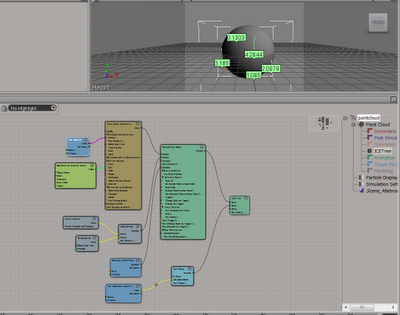## ICE Fundamentals.

### ICE — the Interactive Creative Environment is a visual programming system in Autodesk® Softimage®

#### ICE Attributes

Attributes can be inherent, predefined, or custom.

Inherent

Inherent attributes represent data that is always available in the scene.

Predefined

Predefined attributes are recognized and used by certain basic nodes. However, unlike inherent attributes they are dynamic, meaning that they do not exist until they are set.

Custom

Your ICE tree needs to use a compound that explicitly gets this attribute and uses it in some way.

#### ICE Simulation

Simulated means that the result of the current frame depends on the result of the previous frame rather than on the results of the construction regions that are below it. This is what differentiates a simulation from other types of modifications to an object. For example, if you use a Push deformation on a sphere, at each frame the Push operator gets the undeformed sphere and applies the push. If you move one frame forward, the Push gets the undeformed geometry again and applies the same push.
The only way to modify the push deformation over time is to animate its parameters. However, if the Push operator is in the Simulation region, at each frame it takes the results of the sphere's pushed points from the previous frame and pushes them out more. As you play the simulated push, you see the sphere gradually inflating, without any animation being defined. The only way to return to the undeformed sphere is to go back to the first frame.
When a simulation is active, the regions below the Simulation region are not re-evaluated. This means that if you have any operators in the Animation region, those operators are not evaluated again after the simulation is active. If you want to return to using the animation operators instead of the simulation, simply select and delete the Simulation marker from the stack so that there's no Simulation region.

Simulate Particles Node
The Simulate Particles node is the "standard" particles node that updates the position and velocity of each particle at each frame by doing the following. It:

• Calculates the point accelerations based on mass and force.
• Updates the point velocities based on acceleration.
• Updates the point positions based on velocity.
Sets the simulated frame fraction to 1. The SimulatedFrameFraction attribute defines the fraction of a frame that has already been simulated for a point. Setting this value to 1 prevents points from being resimulated by other Simulate nodes in the same tree (such as the Simulate Rigid Bodies node) or in other trees on the same object.
The Emitter compounds that use this node automatically set the SimulatedFrameFraction to (1 – Age/SimulationStep) so that on its first frame of existence, a particle moves only for a distance proportional to its age. As a result, it appears as if particles have been emitted continuously throughout the frame instead of all at once.

#### ICE Forces

•  Velocity: the speed and direction of an object, or its rate of change in position. This is a vector.
•  Speed: the amplitude of the velocity (the length of the velocity vector) but without the direction that velocity has. This is the ratio of the distance an object moves versus the time it takes.
•  Acceleration: the rate of change of velocity.
For more information on velocity, speed, and acceleration.
•  Force: a push on an object that infers a change in velocity. It is also a vector that indicates the direction of the push. Its length determines the strength of the push.
Force is a vector that gets applied to an object to change its velocity, and the object's mass is taken into consideration (Force = Mass * Acceleration). The Force is calculated by the change in velocity multiplied by the mass.
When the Simulate Particles node applies force to a particle's position, it takes into account the particle mass.
•  Mass: the quantity of matter of an object. The mass determines how quickly objects react to the forces applied to them and how they react in a collision with an obstacle. Objects with a higher mass tend to keep their velocity when exerting forces on them. Therefore, to make a change in an object's motion, you need to apply stronger forces to an object with more mass than to one with little mass.

•  Gravity: the attraction between all objects that have mass. Earth's gravity is a downward acceleration exerted on objects.

•  Drag: a force applied by a fluid on a solid object in the opposite direction to its velocity. The force depends on the velocity of the object and its size, and on the density of the fluid.

ICE Simulation Regions and Nodes
ICE Forces

Here is the Ice Tree we used as an example:*click to make bigger*

And that's it :D

Thanks